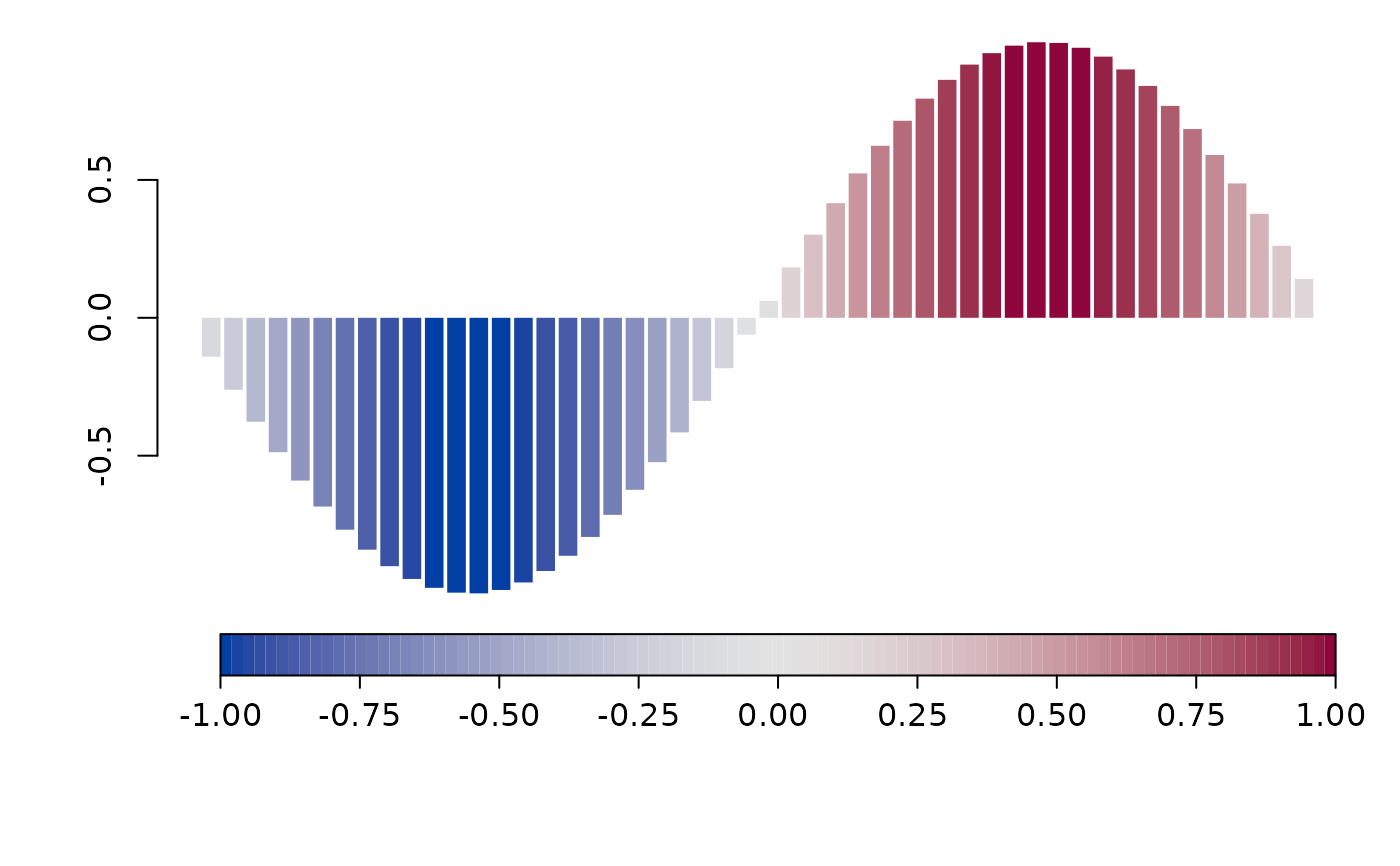Function to generate a color legend, the legend may be added to an existing plot or drawn in a separate plotting window.

colorlegend(color = NULL, ncol = NULL, x = NULL, breaks = NULL,
pos = "center", shift = 0.02, side.legend = 1L, side.ticks = 1L,
range = NULL, lrange = NULL, width = 0.25, height = 0.05,
scale = TRUE, xlim = NULL, ylim = NULL, plot = NULL, full = FALSE,
add = FALSE, col.border = "black", lty.border = 1L, lwd.border = 1L,
ticks = TRUE, at = NULL, col.ticks = "black", lwd.ticks = 1L,
lty.ticks = 1L, length.ticks = 0.3, labels = NULL,
distance.labels = 0, col.labels = "black", cex.labels = 1L,
digits = 2L, swap = FALSE, symmetric = TRUE, xpd = NULL,
title = NULL, side.title = 2, shift.title = c(0, 0),
cex.title = 1, ...)

## Arguments

color

Character, integer. The colors for the legend, may also be a function, e.g. colors = heat.colors.

ncol

Integer, the number of different colors that should be generated if color is a function.

x

Numeric, values for which the color legend should be drawn.

breaks

Numeric, a set of breakpoints for the colors: must give one more breakpoint than ncol.

pos

Character, numeric. The position of the legend. Either a numeric vector, e.g. pos = c(0.1, 0.2) will add the legend at the 10$$\%$$ point in the x-direction and at the 20$$\%$$ point in the y-direction of the plotting window, may also be negative, or one of the following: "bottomleft", "topleft", "topright", "bottomright", "left", "right", "top", "bottom" and "center".

shift

Numeric, if argument pos is a character, shift determines the distance of the legend from the plotting box.

side.legend

Integer, if set to 2 the legend will be flipped by 90 degrees.

side.ticks

Integer, if set to 2, the ticks and labels will be on the opposite site of the legend.

range

Numeric, specifies a range for x values for which the legend should be drawn.

lrange

Numeric, specifies the range of legend.

width

Numeric, the width of the legend, if scale = TRUE the width is proportional to the x-limits of the plotting window.

height

Numeric, the height of the legend, if scale = TRUE the height is proportional to the y-limits of the plotting window.

scale

Logical, if set to TRUE, the width and height of the legend will be calculated proportional to the x- and y-limits of the plotting window.

xlim

Numeric, the x-limits of the plotting window the legend should be added for, numeric vector, e.g., returned from function range.

ylim

Numeric, the y-limits of the plotting window the legend should be added for, numeric vector, e.g., returned from function range.

plot

Logical, if set to TRUE, the legend will be drawn in a separate plotting window.

full

Logical, if set to TRUE, the legend will be drawn using the full window range.

Logical, if set to TRUE, the legend will be added to an existing plot.

col.border

The color of the surrounding border line of the legend.

lty.border

The line type of the surrounding border line of the legend.

lwd.border

The line width of the surrounding border line of the legend.

ticks

Logical, if set to TRUE, ticks will be added to the legend.

at

Numeric, specifies at which locations ticks and labels should be added.

col.ticks

The colors of the ticks.

lwd.ticks

The line width of the ticks.

lty.ticks

The line type of the ticks.

length.ticks

Numeric, the length of the ticks as percentage of the height or width of the colorlegend.

labels

Character, specifies labels that should be added to the ticks.

distance.labels

Numeric, the distance of the labels to the ticks, proportional to the length of the ticks.

col.labels

The colors of the labels.

cex.labels

Text size of the labels.

digits

Integer, the decimal places if labels are numerical.

swap

Logical, if set to TRUE colors will be represented in reverse order.

symmetric

Logical, if set to TRUE, a symmetric legend will be drawn corresponding to the +- max(abs(x)) value.

xpd

Sets the xpd parameter in function par.

title

Character, a title for the legend.

side.title

Integer, 1 or 2. Specifies where the legend is placed, either on top if side.title = 1 or at the bottom if side.title = 2.

shift.title

Numeric vector of length 2. Specifies a possible shift of the title in either x- or y-direction.

cex.title

Text size for the title.

...

Other graphical parameters to be passed to function text.

## Value

A named list with the colors generated, the breaks and the function map, which may be used for mapping of x values to the colors specified in argument colors, please see the examples below.

## Examples

## Play with colorlegend.
colorlegend()colorlegend(side.legend = 2)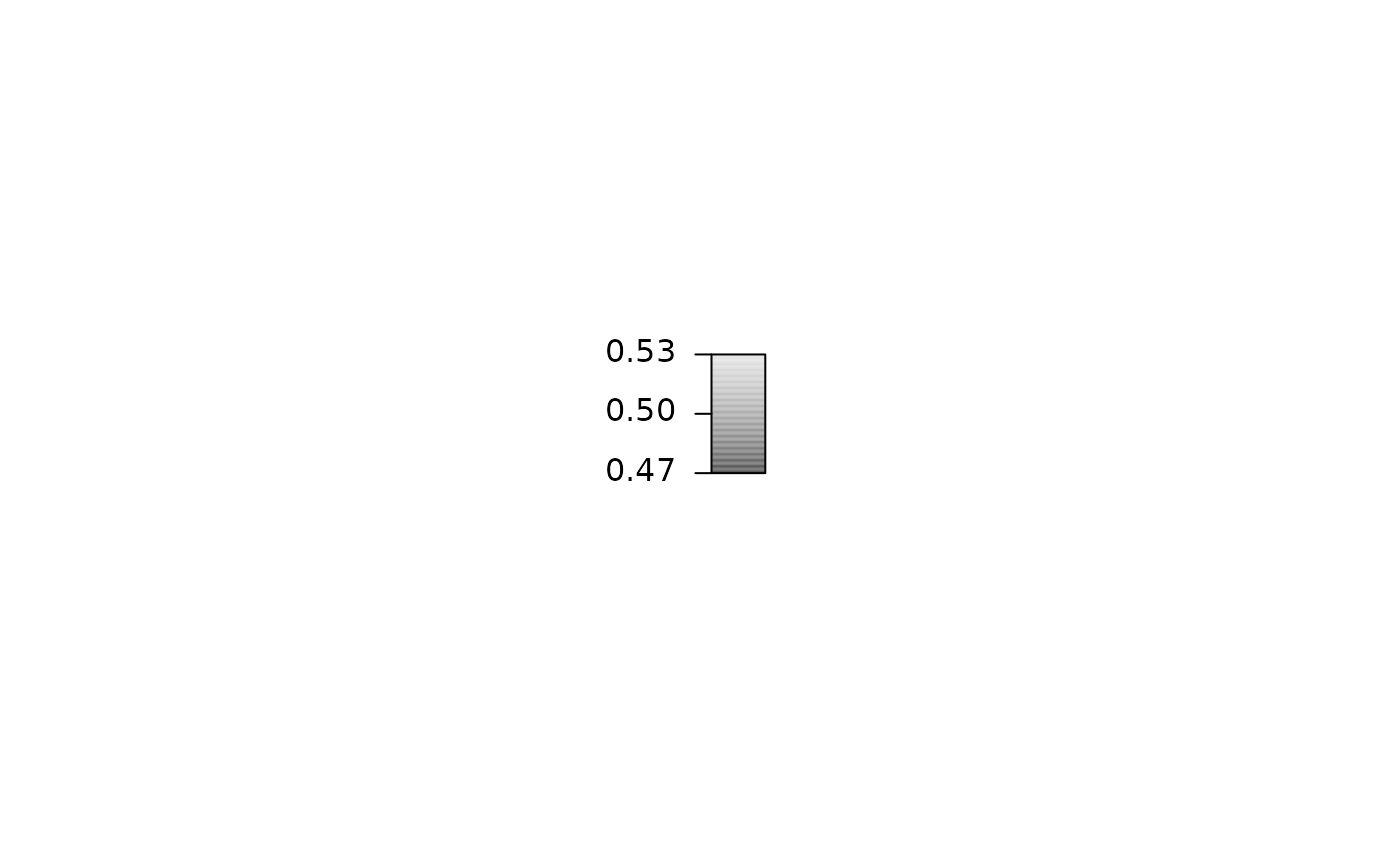colorlegend(side.legend = 2, side.ticks = 2)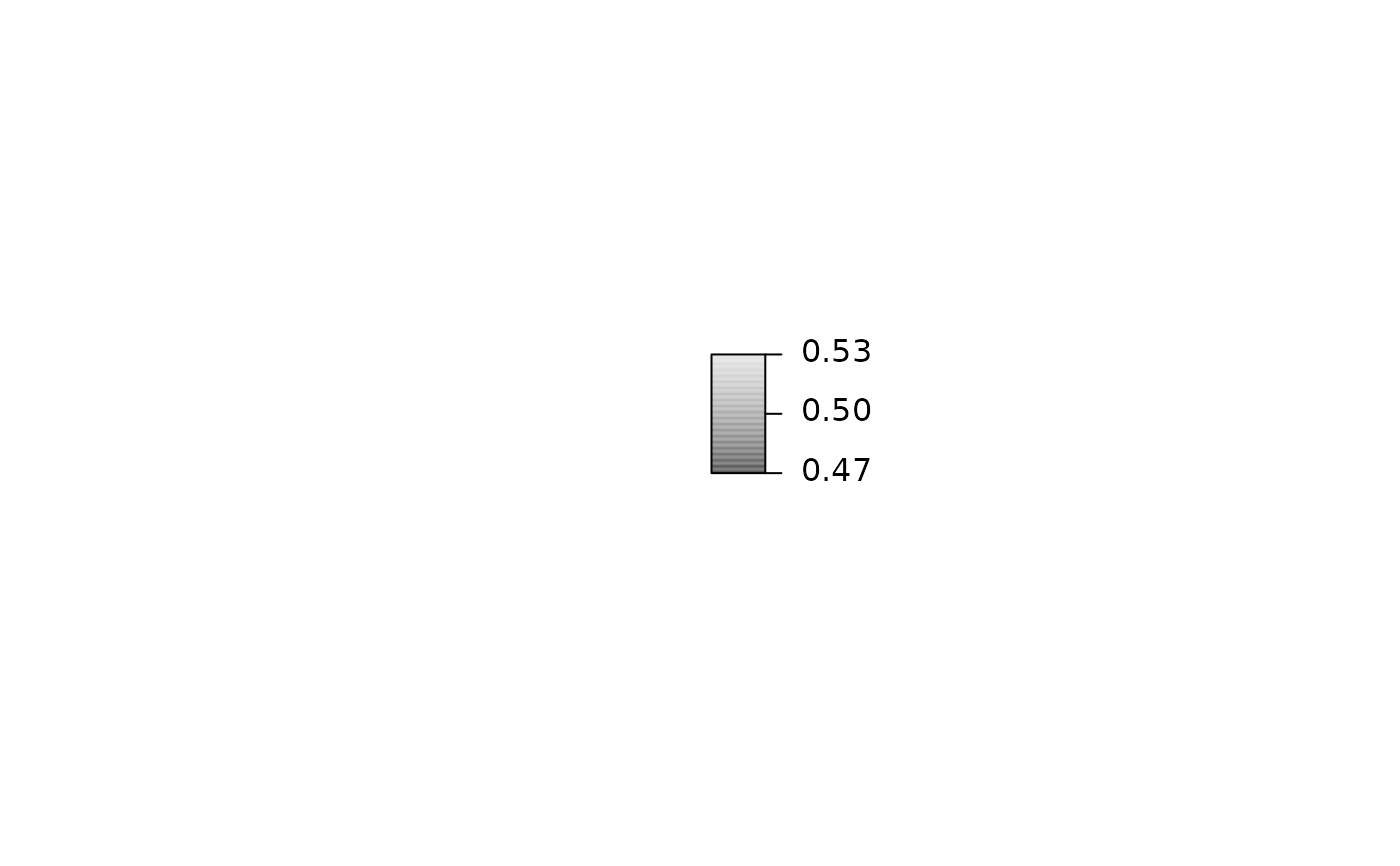colorlegend(height = 2)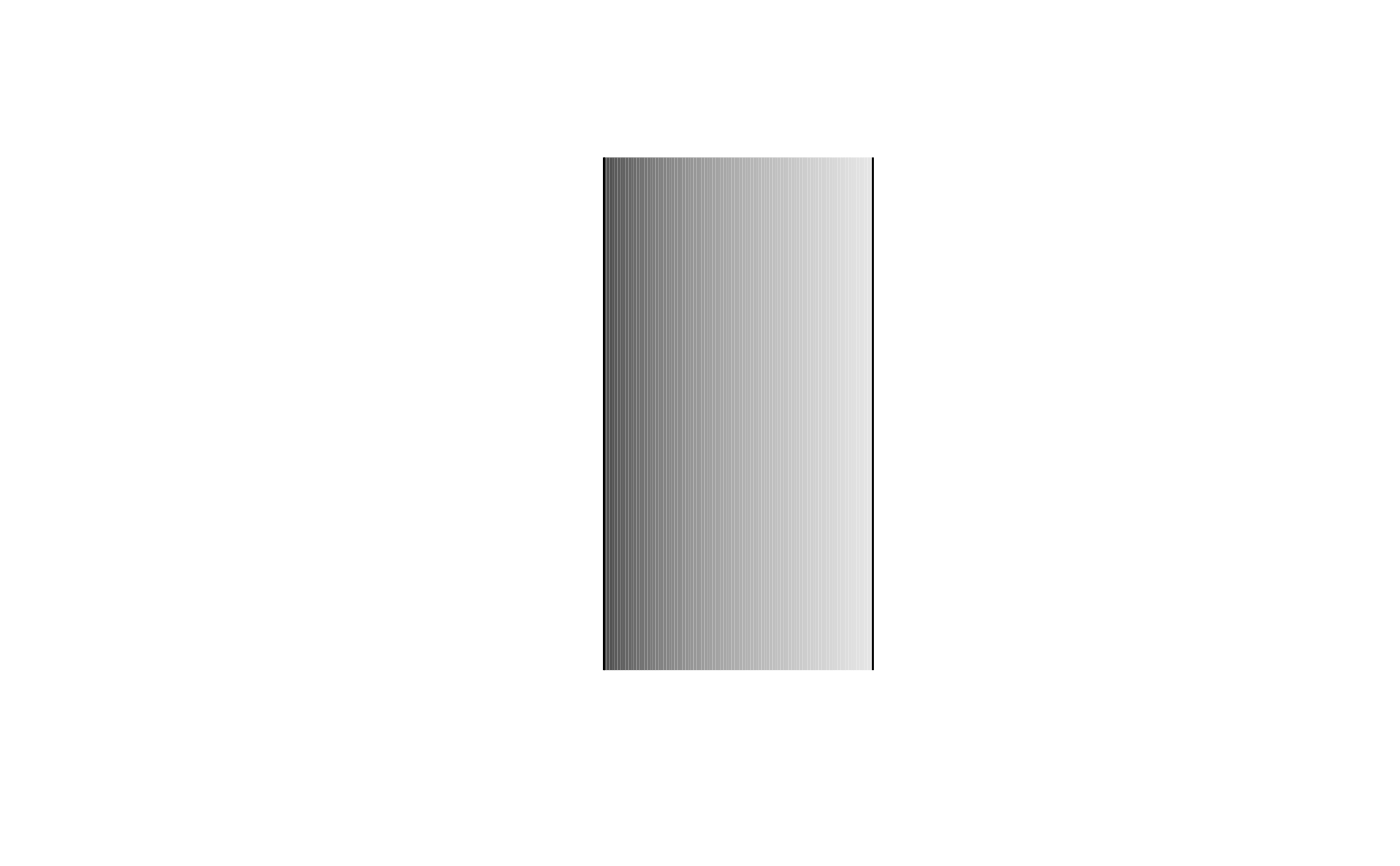colorlegend(width = 1, height = 0.8, scale = FALSE,
pos = c(0, 0.2), length.ticks = 0.5)colorlegend(color = heat.colors, ncol = 9)colorlegend(color = heat.colors, ncol = 9, swap = TRUE)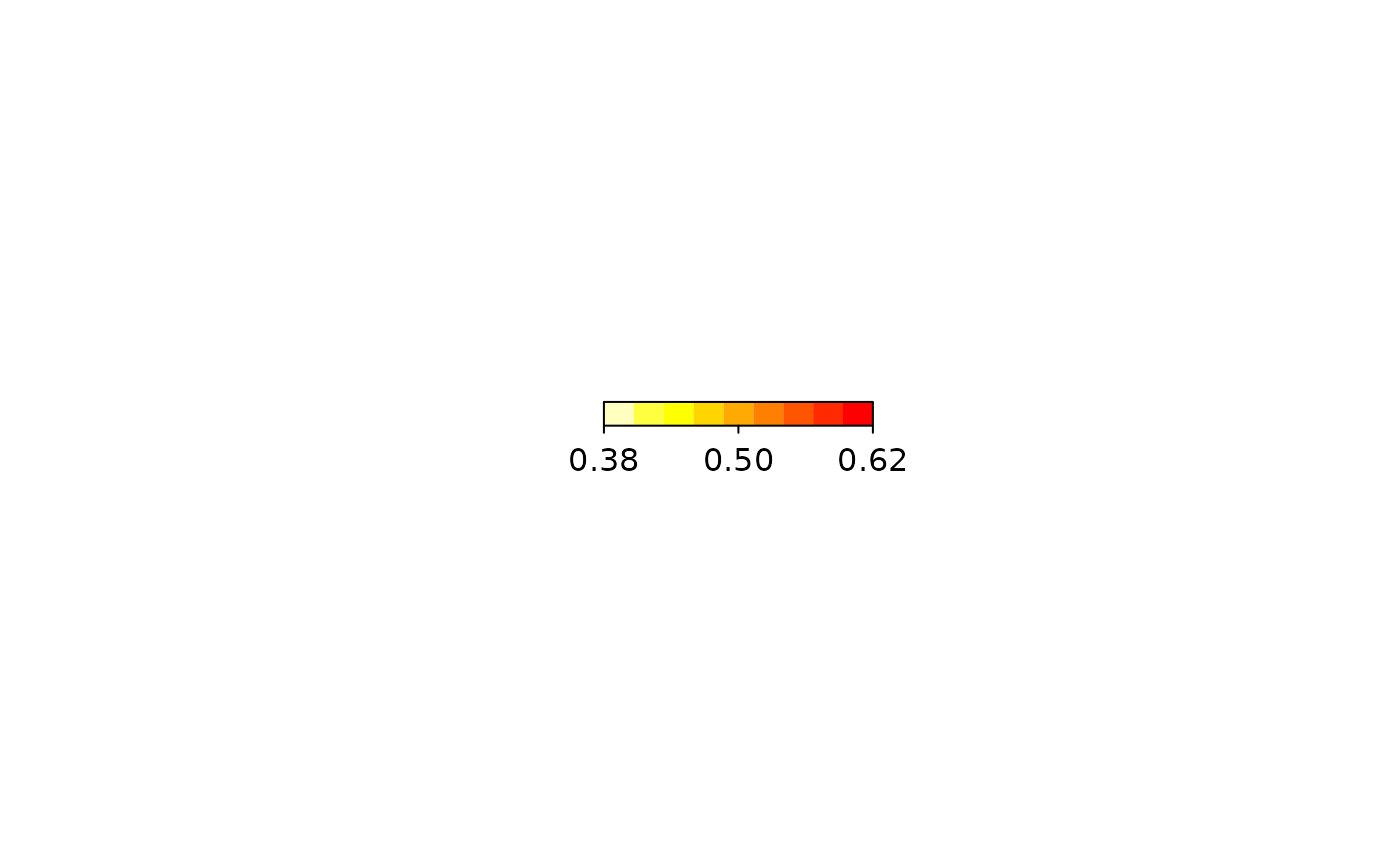colorlegend(pos = "bottomleft")colorlegend(pos = "topleft")colorlegend(pos = "topright")colorlegend(pos = "bottomright")## Take x values for the color legend.
x <- runif(100, -2, 2)
colorlegend(color = diverge_hcl, x = x)colorlegend(color = diverge_hcl, x = x, at = c(-1.5, 0, 1.5))colorlegend(color = diverge_hcl, x = x, at = c(-1.5, 0, 1.5),
labels = c("low", "middle", "high"))colorlegend(color = rainbow_hcl, x = x, at = c(-1.5, 0, 1.5),
labels = c("low", "middle", "high"), length.ticks = 1.5)colorlegend(color = heat_hcl, x = x, at = c(-1.5, 0, 1.5),
labels = c("low", "middle", "high"), length.ticks = 1.5,
lwd.border = 2, lwd.ticks = 2, cex.labels = 1.5, font = 2)colorlegend(color = topo.colors, x = x, at = c(-1.5, 0, 1.5),
labels = c("low", "middle", "high"), length.ticks = 1.5,
lwd.border = 2, lwd.ticks = 2, cex.labels = 1.5, font = 2,
col.border = "green3", col.ticks = c(2, 5, 2),
col.labels = c(6, 4, 3))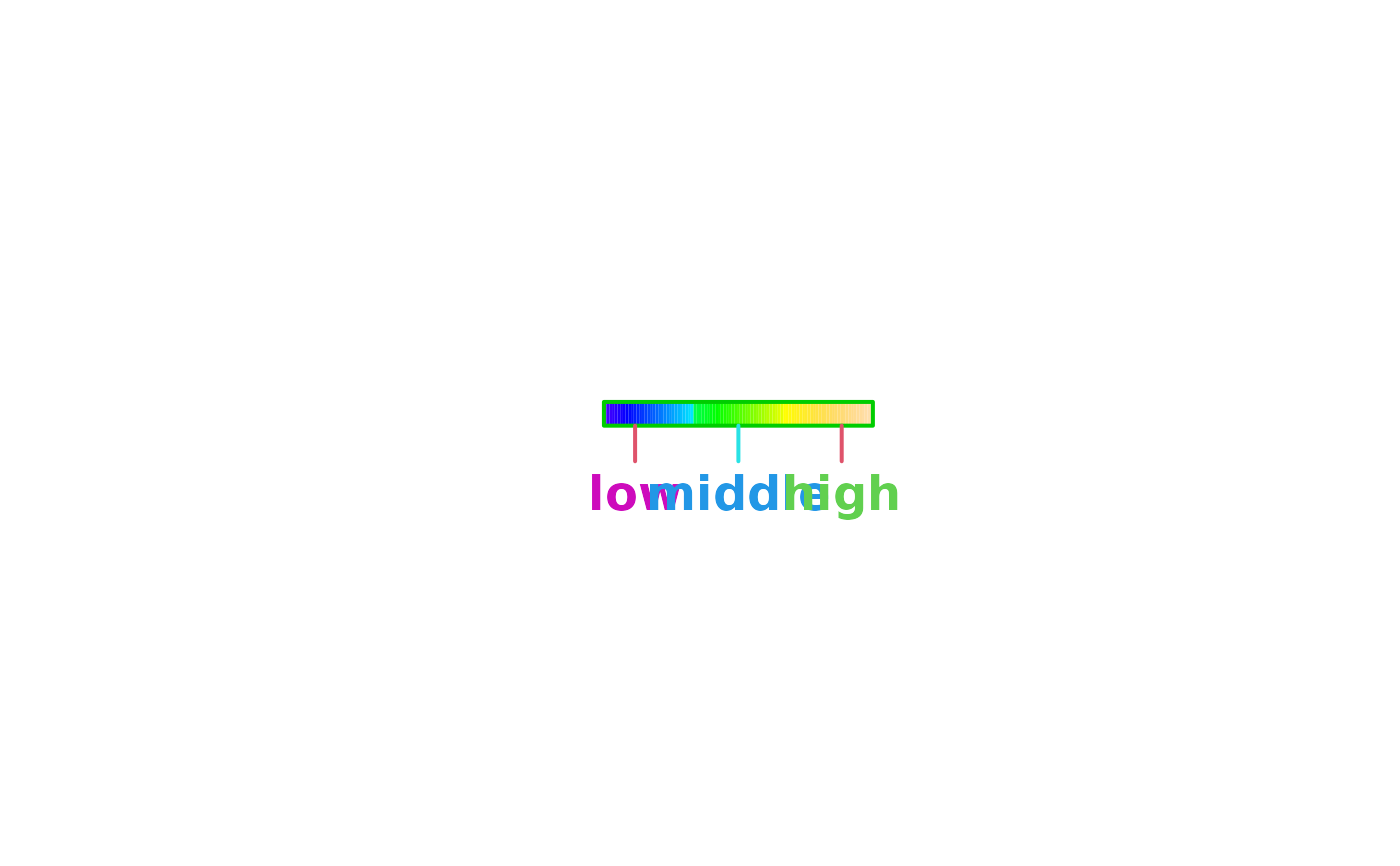colorlegend(color = diverge_hsv, x = x, at = c(-1.5, 0, 1.5),
labels = c("low", "middle", "high"), length.ticks = 1.5,
lwd.border = 2, lwd.ticks = 2, cex.labels = 1.5, font = 2,
col.border = "green3", col.ticks = c(2, 5, 2),
col.labels = c(6, 4, 3), lty.border = 2, lty.ticks = c(2, 3, 2))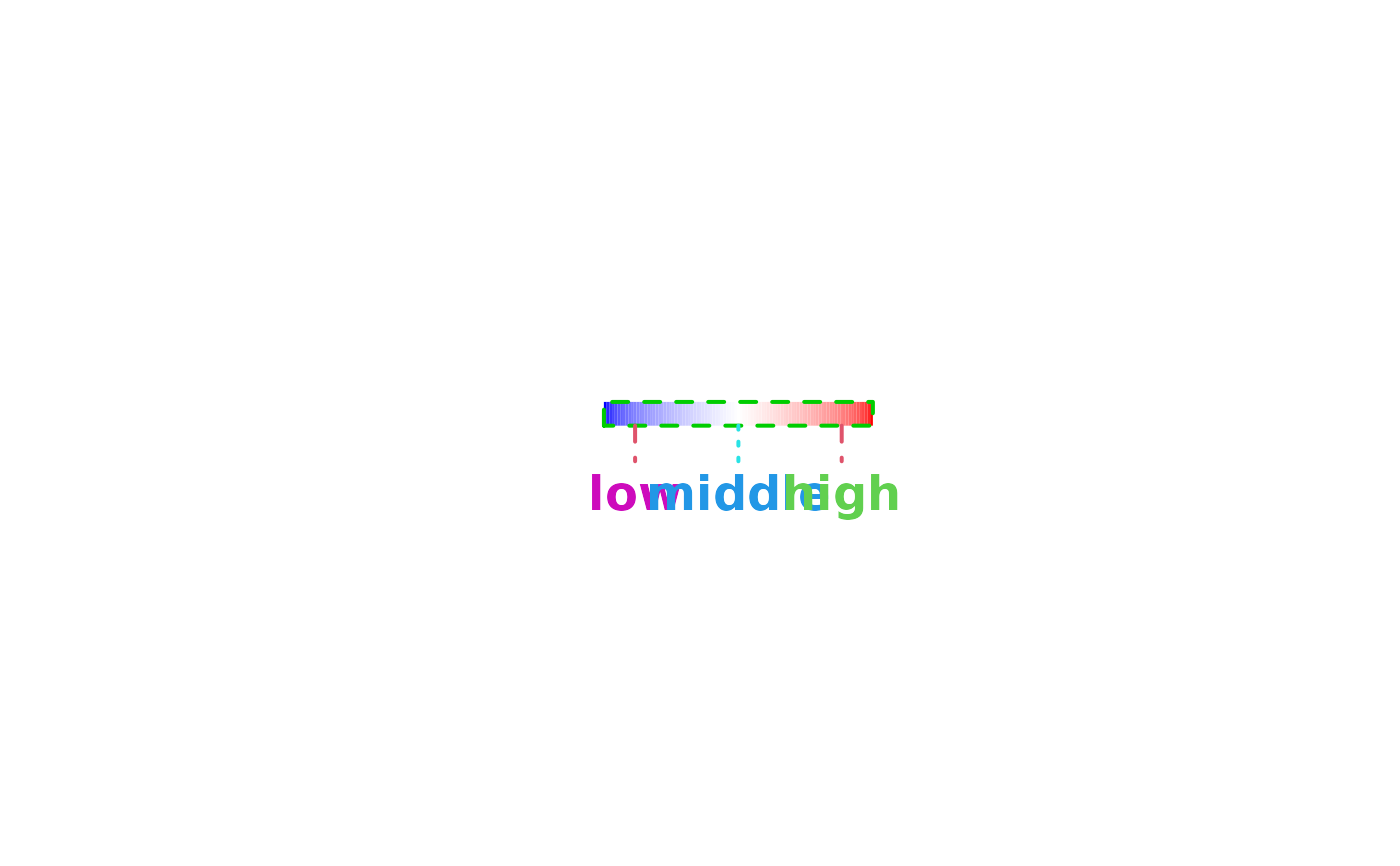colorlegend(color = diverge_hsv, x = x, at = c(-1.5, 0, 1.5),
labels = c("low", "middle", "high"), length.ticks = 1.5,
lwd.border = 2, lwd.ticks = 2, cex.labels = 1.5, font = 2,
col.border = "green3", col.ticks = c(2, 5, 2),
col.labels = c(6, 4, 3), lty.border = 2, lty.ticks = c(2, 3, 2),
ncol = 3)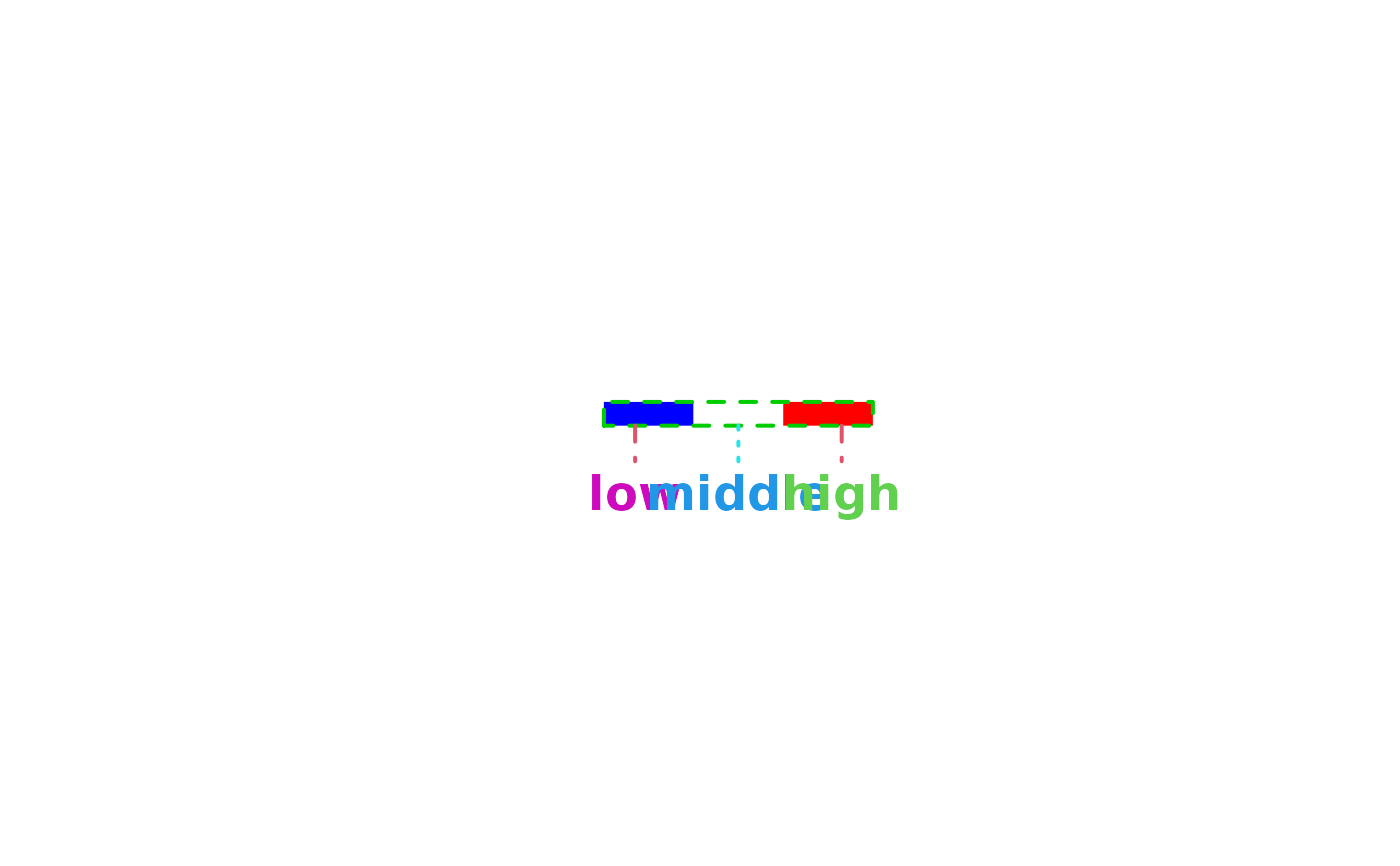colorlegend(color = c("red", "white", "red"), x = x, at = c(-1.5, 0, 1.5),
labels = c("low", "middle", "high"), length.ticks = 1.5,
lwd.border = 2, lwd.ticks = 2, cex.labels = 1.5, font = 2,
col.border = "green3", col.ticks = c(2, 5, 2),
col.labels = c(6, 4, 3), lty.border = 2, lty.ticks = c(2, 3, 2),
ncol = 3, breaks = c(-2, -1, 1, 2))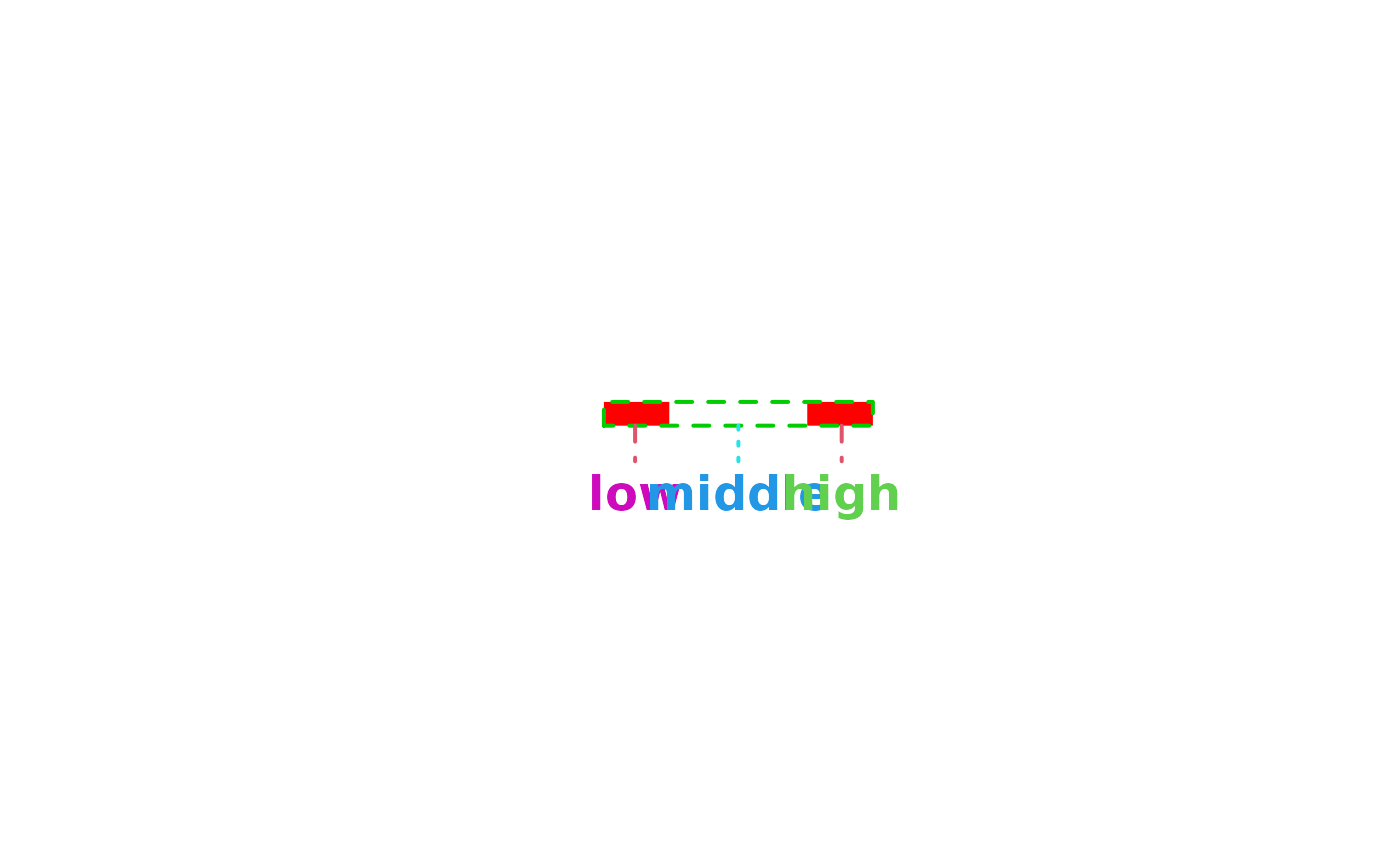colorlegend(color = diverge_hcl, x = x, range = c(-3, 3))colorlegend(color = diverge_hcl, x = x, range = c(-3, 3), lrange = c(-6, 6))## Combine plot with color legend.
n <- 100
x <- y <- seq(-3, 3, length.out = n)
z <- outer(sin(x), cos(x))
pal <- colorlegend(color = diverge_hcl, x = z, plot = FALSE)
par(mar = c(4.1, 4.1, 1.1, 1.1))
layout(matrix(c(1, 2), nrow = 1), widths = c(1, 0.3))
image(x = x, y = y, z = z, col = pal$colors, breaks = pal$breaks)
par(mar = c(4.1, 0.1, 1.1, 3.1))
colorlegend(color = diverge_hcl, x = z, plot = TRUE, full = TRUE,
side.legend = 2, side.ticks = 2)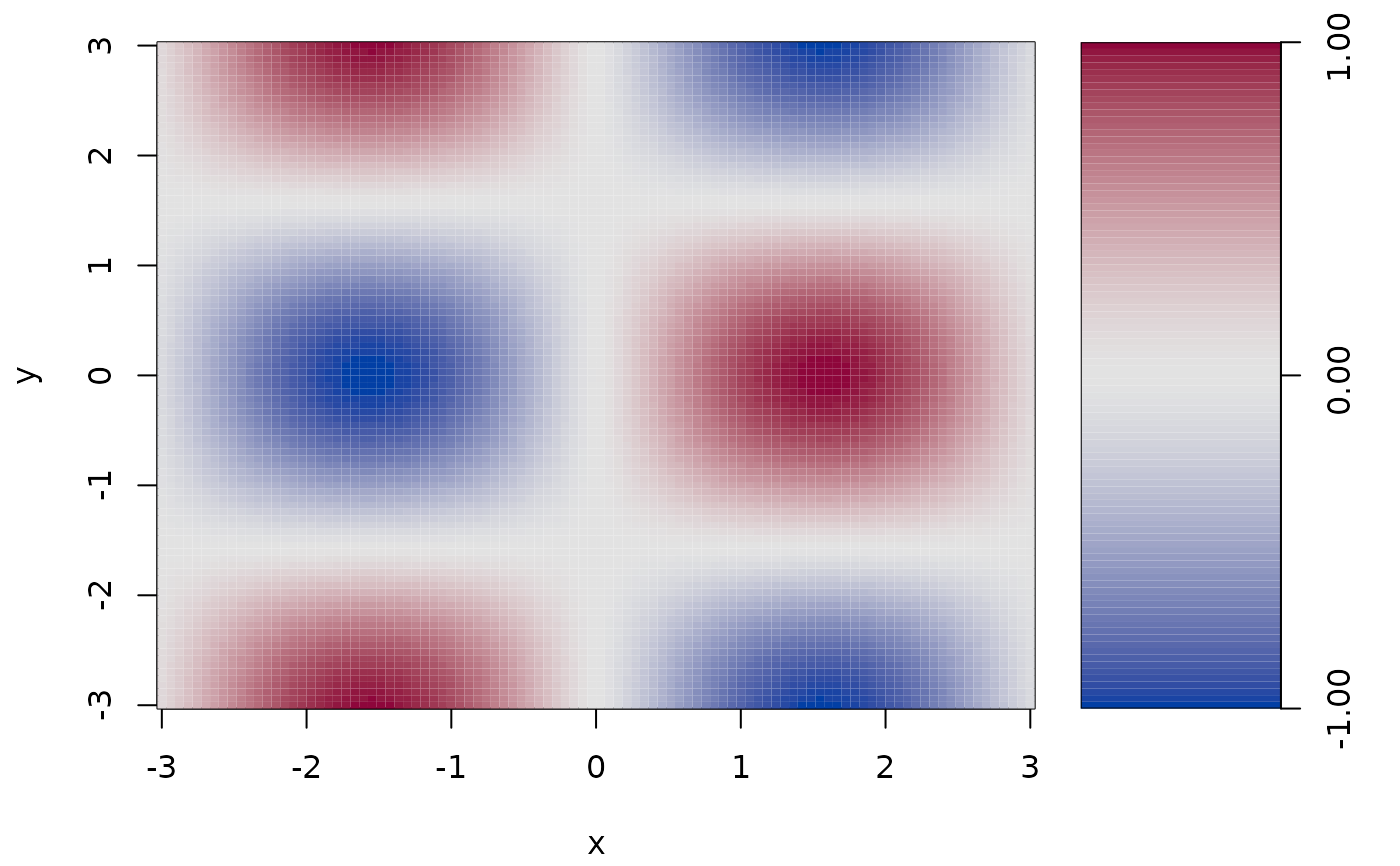par(op)

## Another example with different plot.
n <- 50
x <- sin(seq(-3, 3, length.out = n))
pal <- colorlegend(color = diverge_hcl, x = x, plot = FALSE)